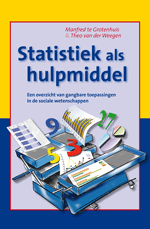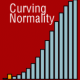# Book: A conceptual introduction to statisticsHow does one teach statistics? Is it more important to start with mathematical thoroughness, or to help students to gain a conceptual understanding first? There’s something to say about both, depending on the setting you’re in, but fact is that most books on statistics (even the introductory ones) rapidly delve into the mathematical depths of inferential statistics. Few give a comprehensive introduction to statistics for those without the otherwise indispensable mathematical background. Manfred te Grotenhuis and Theo van der Weegen recently published an introductory book on statistics called Statistics as a Tool” (Statistiek als Hulpmiddel, in Dutch), explaining statistical concepts using words and graphs, rather than formulas.

I have had the pleasure of providing minor assistance to this book, so please let me introduce it here. Admittedly I’m not impartial on this one, but I sincerely believe this book to be an asset to introductory statistics courses and those who have to interpret or perform statistics, without the need of knowing all about the mathematical niceties.

With the focus on practical application rather than statistical theory, the first chapter starts explaining the goal of inferential statistics, meanwhile introducing the concepts of measurement and variables. Considerable attention is paid to the importance of high quality data to perform your analyses on. The second chapter deals with descriptive statistics, both in a numerical and a graphical way. Here, also the concepts of a distribution and of correlation are introduced. The third and final chapter discusses the testing of hypotheses, using techniques as the cross-table, tests for means and proportions, various forms of correlation, and finally multiple regression.

Clearly, the setup of this book is what one might expect from an introduction to statistics. However, I think this book has a unique approach by its strong focus on the conceptual level, rather than the (mathematical) statistical theory. Nevertheless, it does not shy away from relatively complex subjects such as the multiple regression. Even on the conceptual level, it pays a lot of attention to the assumptions required for the various analyses discussed. The practical approach of this book is enhanced even further, because all examples come from ‘real life’ research. On the accompanying website SPSS data files and syntax files are made available, so that every example from the book can be repeated by the reader.

Aimed at the novice statistics student, this book offers a comprehensible and conceptual approach at statistics. It will surely help students of statistics to grasp what they’re actually doing when pushing SPSS’s buttons or trying to interpret published figures. In that sense, I think that for many statistics student, this book successfully reaches is goal of transforming statistics form an abstract undertaking to an actually useful and applicable tool.

## 7 comment on “Book: A conceptual introduction to statistics”

•Thanks Rense,

I’m not that good at math (to put it mildly) but everso more interested in scientific research. Since this book is cheap, and I’m a (nursing) student, I’ll think I’ll give it a go!

•Lovely! Glad to be of service. Since I’ve been a nursing student myself once, I can really imagine how this book helps you in understanding statistics better ‘from the bottom up’, and how this understanding might help in your profession.
Be sure to report back your experiences with the book, if you’d like.

•Is it me, or is naming the nursing profession causally connected with an explanation containing the word ‘bottom’ in a reply? (p<.0001) 😀

•Correlation does not prove causality

•Correlation is a first hint for causality though. Besides, in this case, also the second criterion for “proving” causality was satisfied. That is, Bram first mentioned the nursing profession and after that Rense replied with a comment containing the word “bottom”…of course, there still might be third variables in play

•Manfred te Grotenhuis